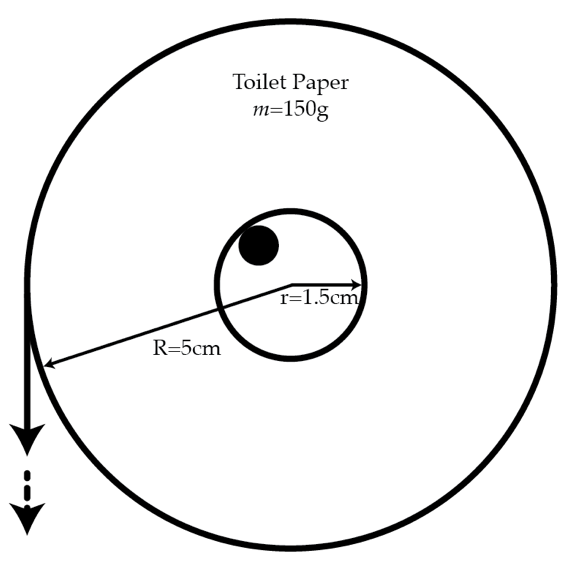# The physics of toilet paper

A toilet paper roll of mass $m=150~\mbox{g}$ with inner radius $r=1.5~\mbox{cm}$ and outer radius $R=5~\mbox{cm}$ is put on a small cylindrical bar with coefficient of friction $k=2$. If we pull the paper straight down very slowly and increase the force very gradually, the inner part of the roll will first move without slipping on the bar. Then at some critical force $F_c$, it will start to slip. Assume that the pulled out piece of paper is always vertical. Find $F_c$ in Newtons.Details and assumptions

• The gravitational acceleration is $g=-9.8~\mbox{m/s}^2$
×

Problem Loading...

Note Loading...

Set Loading...